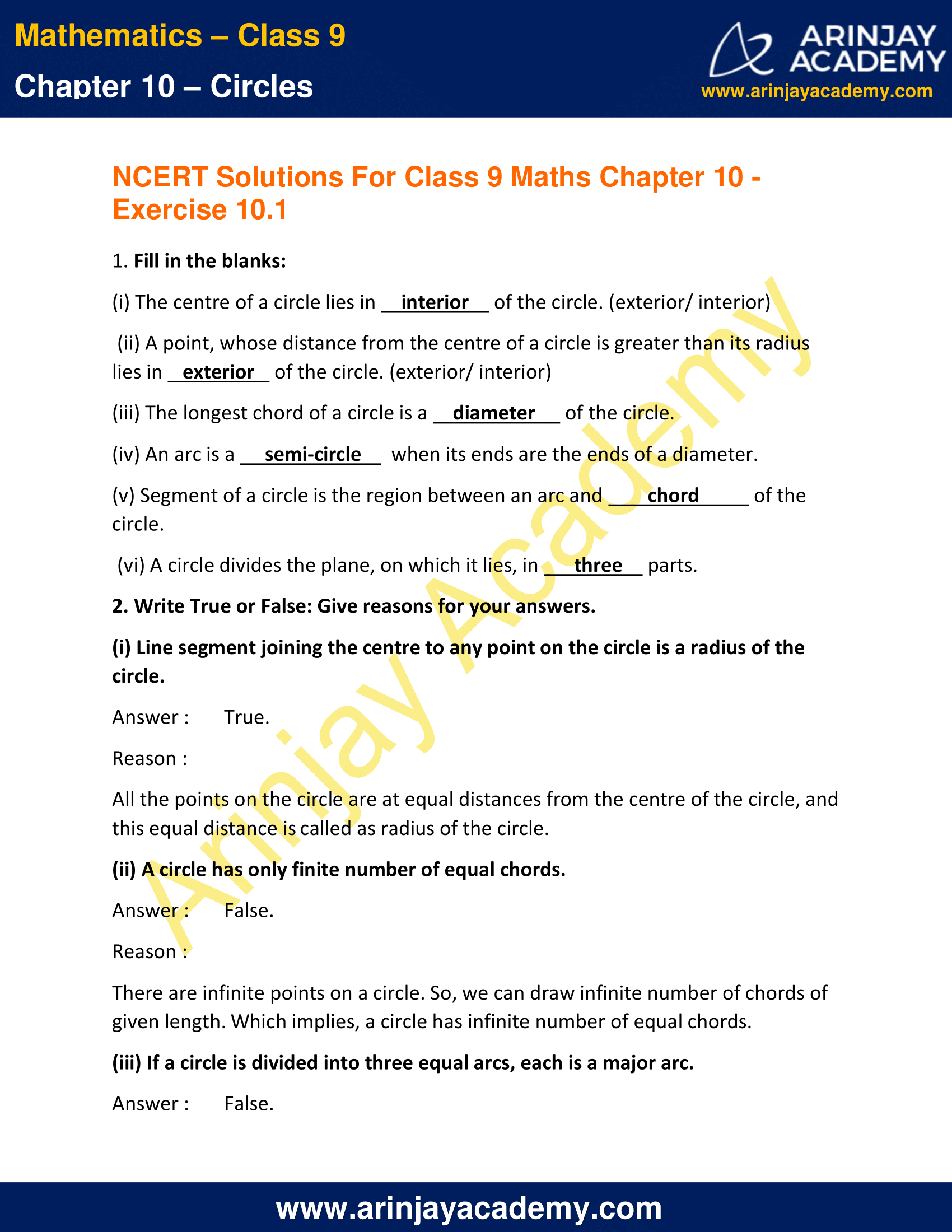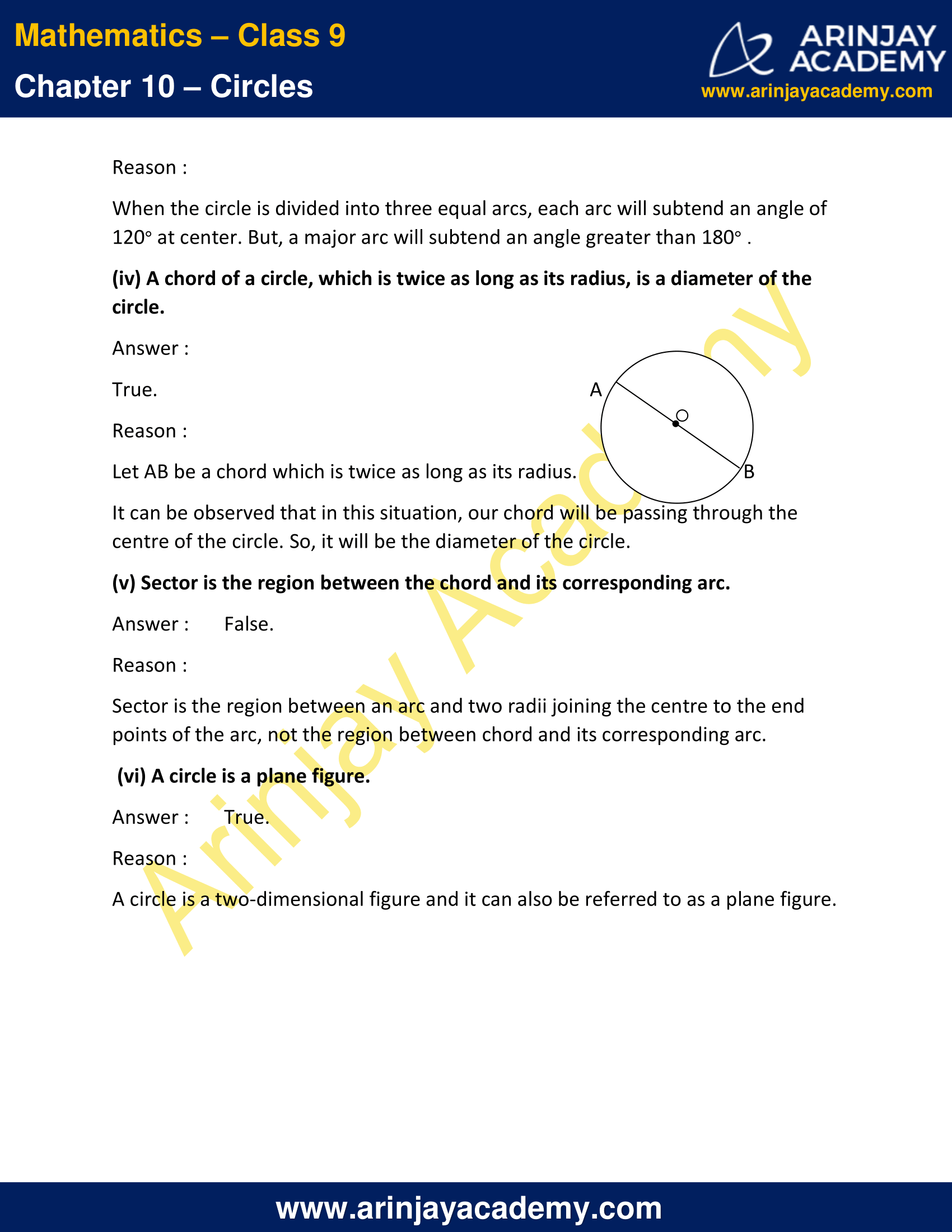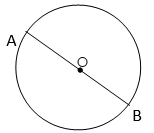# NCERT Solutions for Class 9 Maths Chapter 10 Exercise 10.1 – Circles

Download NCERT Solutions for Class 9 Maths Chapter 10 Exercise 10.1 – Circles. This Exercise contains 2 questions, for which detailed answers have been provided in this note. In case you are looking at studying the remaining Exercise for Class 9 for Maths NCERT solutions for Chapter 10 or other Chapters, you can click the link at the end of this Note.

### NCERT Solutions for Class 9 Maths Chapter 10 Exercise 10.1 – CirclesNCERT Solutions for Class 9 Maths Chapter 10 Exercise 10.1 – Circles

1. Fill in the blanks:

(i) The centre of a circle lies in     interior     of the circle. (exterior/ interior)

(ii) A point, whose distance from the centre of a circle is greater than its radius lies in    exterior    of the circle. (exterior/ interior)

(iii) The longest chord of a circle is a  diameter  of the circle.

(iv) An arc is a semi-circle  when its ends are the ends of a diameter.

(v) Segment of a circle is the region between an arc and chord of the circle.

(vi) A circle divides the plane, on which it lies, in three parts.

(i) Line segment joining the centre to any point on the circle is a radius of the circle.

Reason : All the points on the circle are at equal distances from the centre of the circle, and this equal distance is called as radius of the circle.

(ii) A circle has only finite number of equal chords.

Reason : There are infinite points on a circle. So, we can draw infinite number of chords of given length. Which implies, a circle has infinite number of equal chords.

(iii) If a circle is divided into three equal arcs, each is a major arc.

Reason : When the circle is divided into three equal arcs, each arc will subtend an angle of 120° at center. But, a major arc will subtend an angle greater than 180° .

(iv) A chord of a circle, which is twice as long as its radius, is a diameter of the circle.Reason : Let AB be a chord which is twice as long as its radius.

It can be observed that in this situation, our chord will be passing through the centre of the circle. So, it will be the diameter of the circle.

(v) Sector is the region between the chord and its corresponding arc.

Reason : Sector is the region between an arc and two radii joining the centre to the end points of the arc, not the region between chord and its corresponding arc.

(vi) A circle is a plane figure.